# R S Aggarwal Solutions for Class 10 Maths Exercise 18E Chapter 18 Mean, Median, Mode of Grouped Data, Cumulative Frequency Graph and Ogive

In this section, students will get answers for all the questions enlisted under exercise 18E. R S Aggarwal Solutions for this exercise mentioned below are solved by subject experts using step by step problem solving approach. Students can download Class 10 Maths Chapter 18 R S Aggarwal Solutions and score well in exams.

## Download PDF of R S Aggarwal Solutions for Class 10 Maths Chapter 18 Mean, Median, Mode of Grouped Data, Cumulative Frequency Graph and Ogive Exercise 18E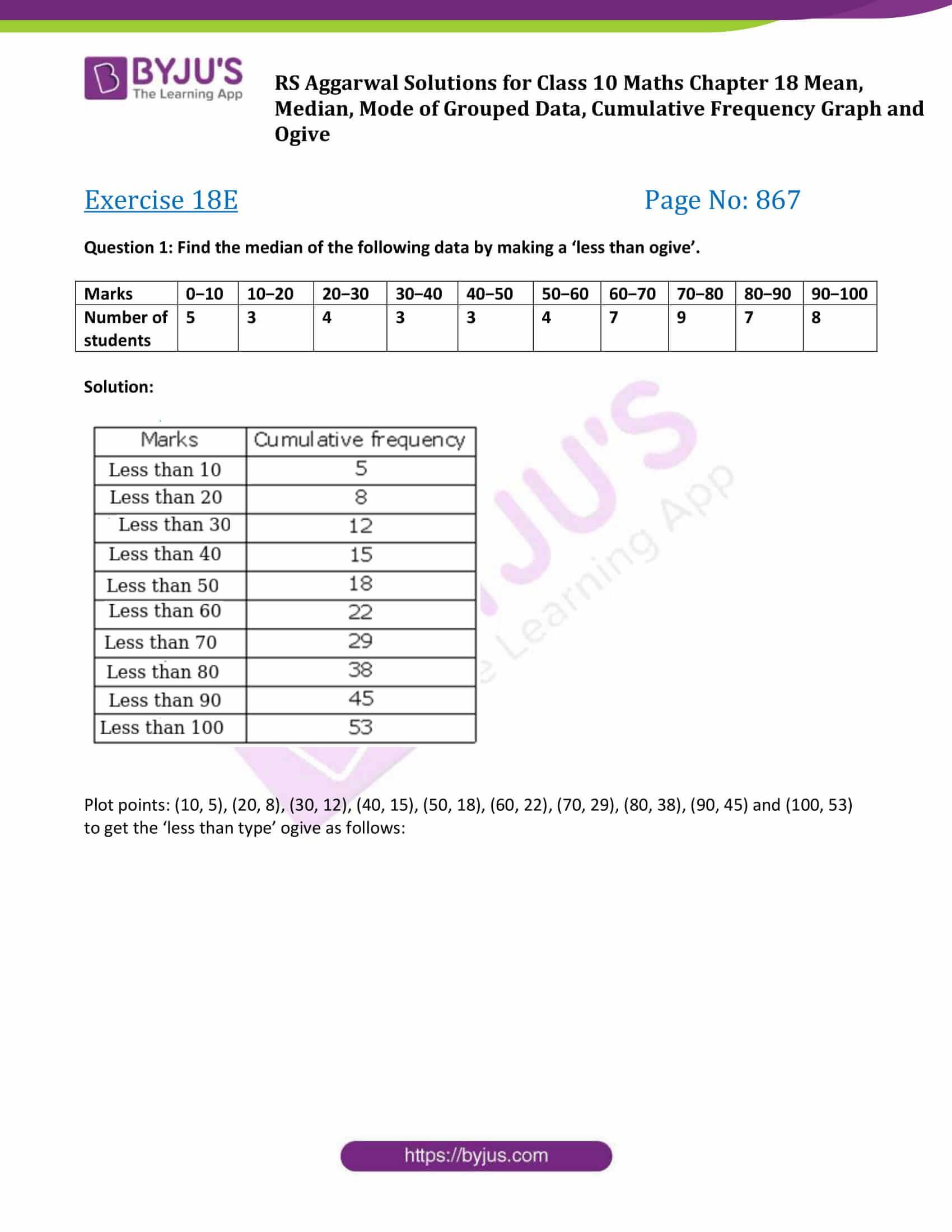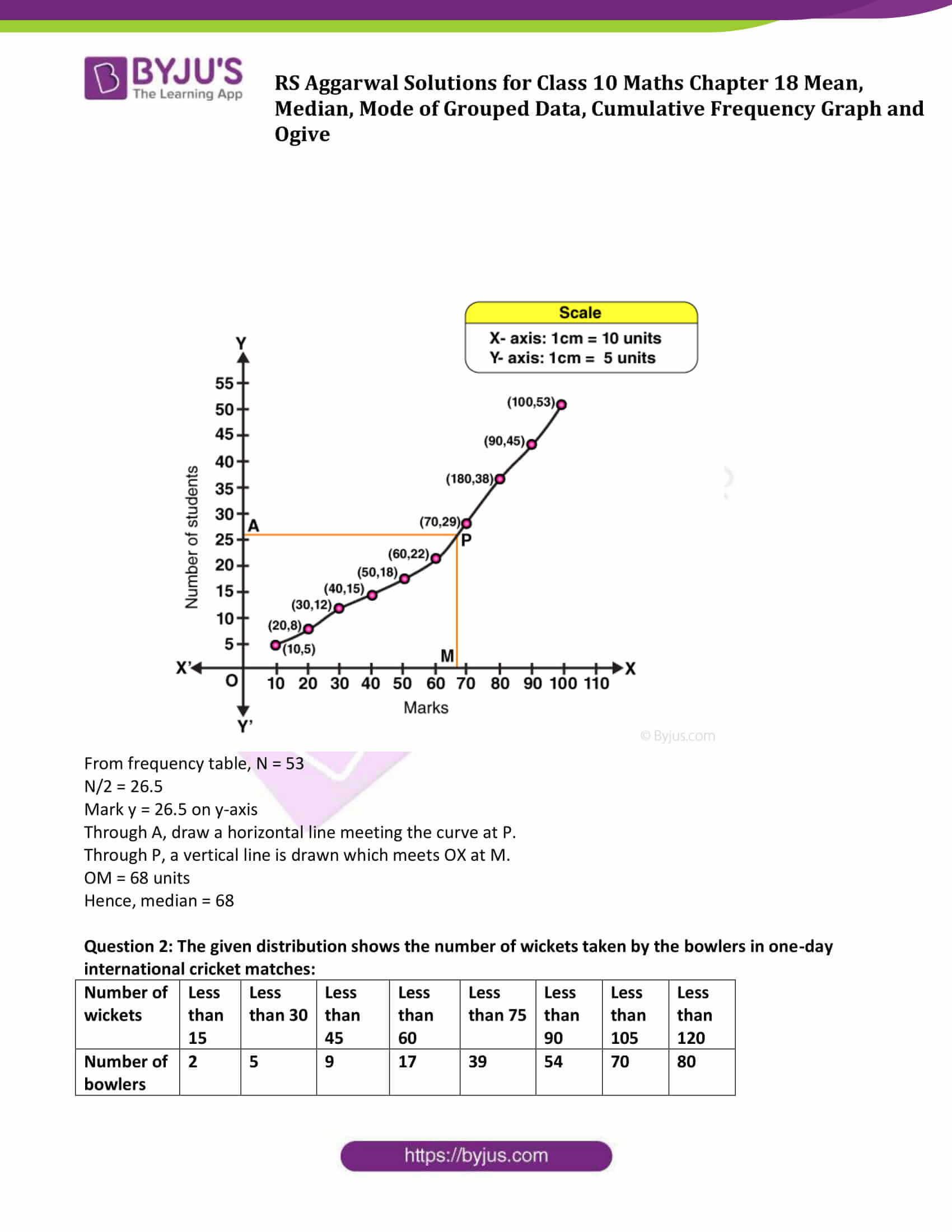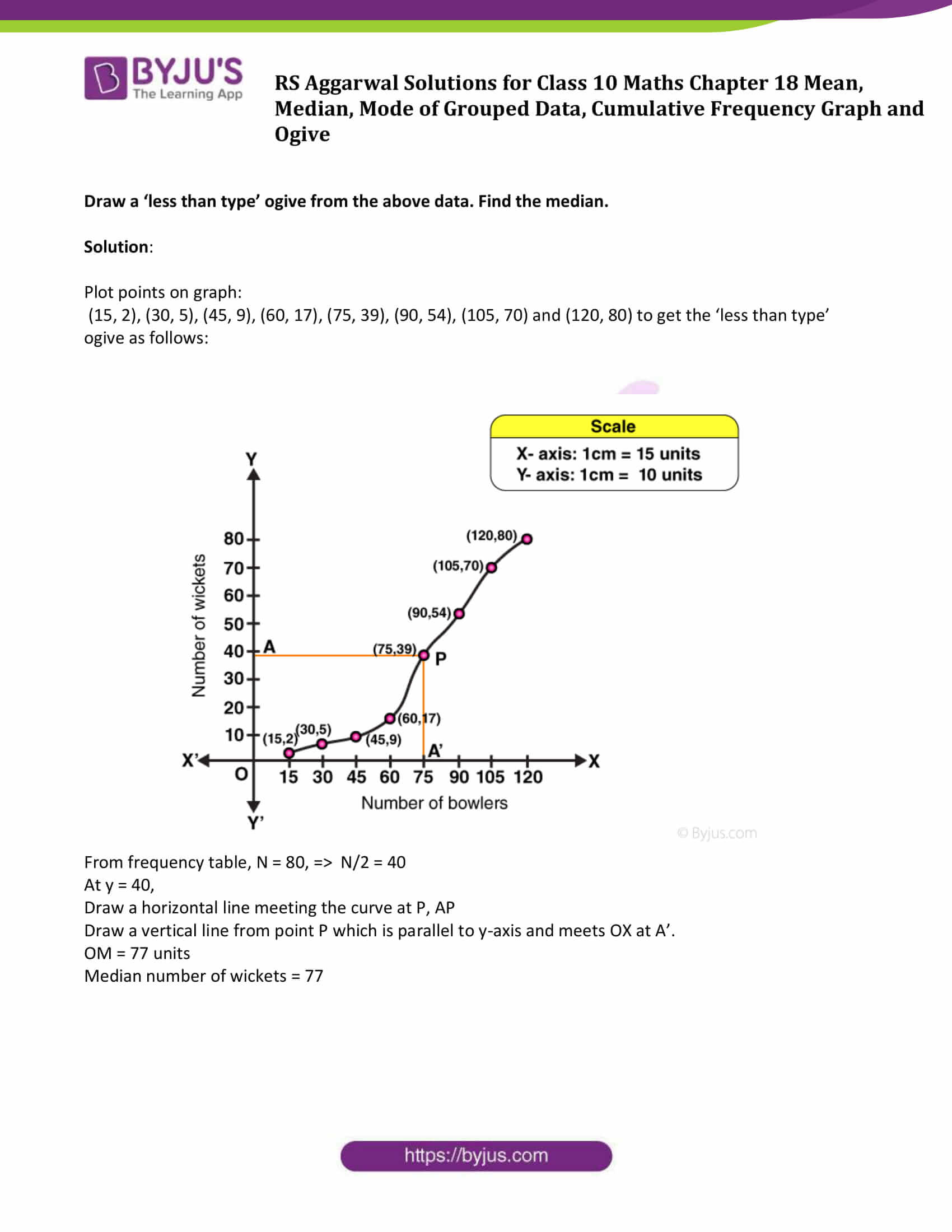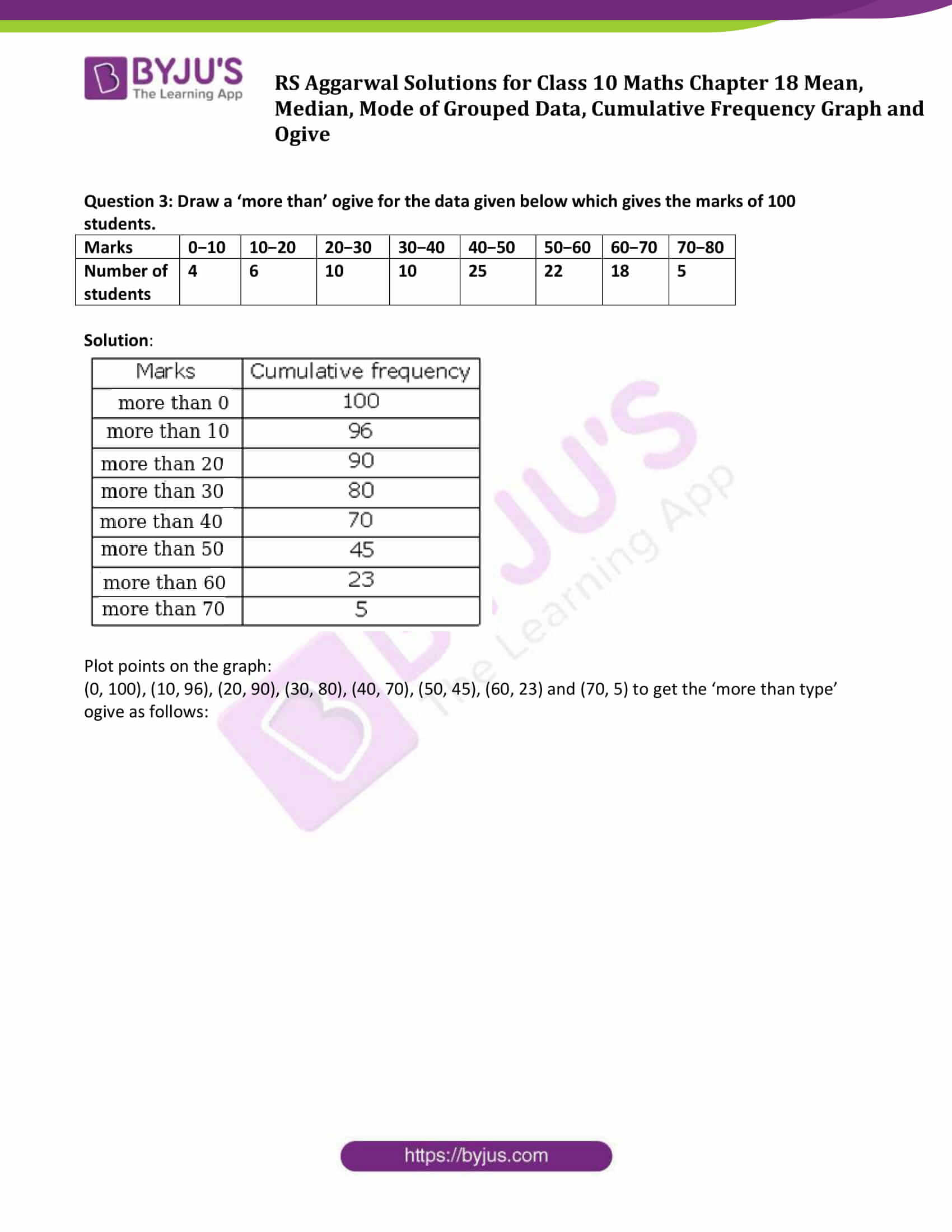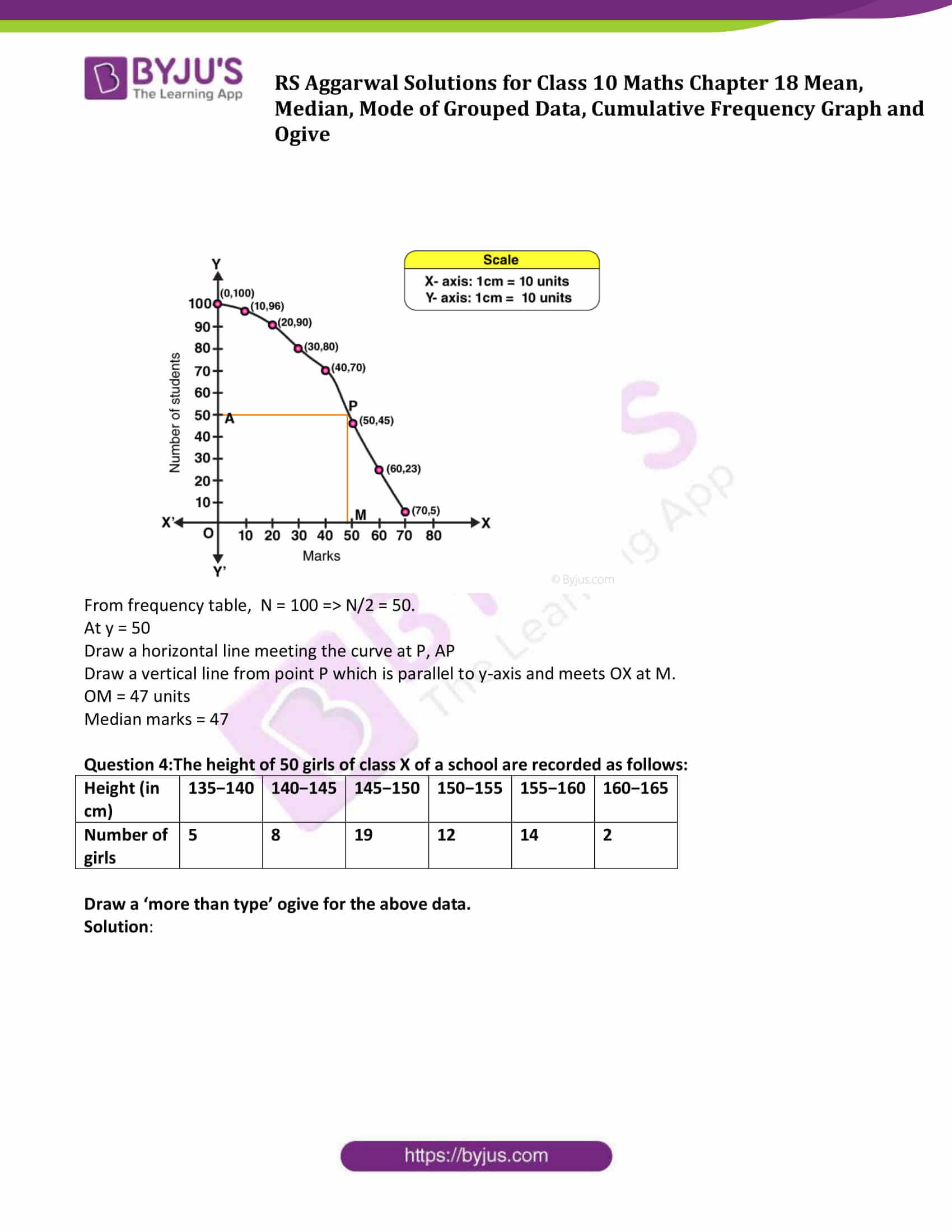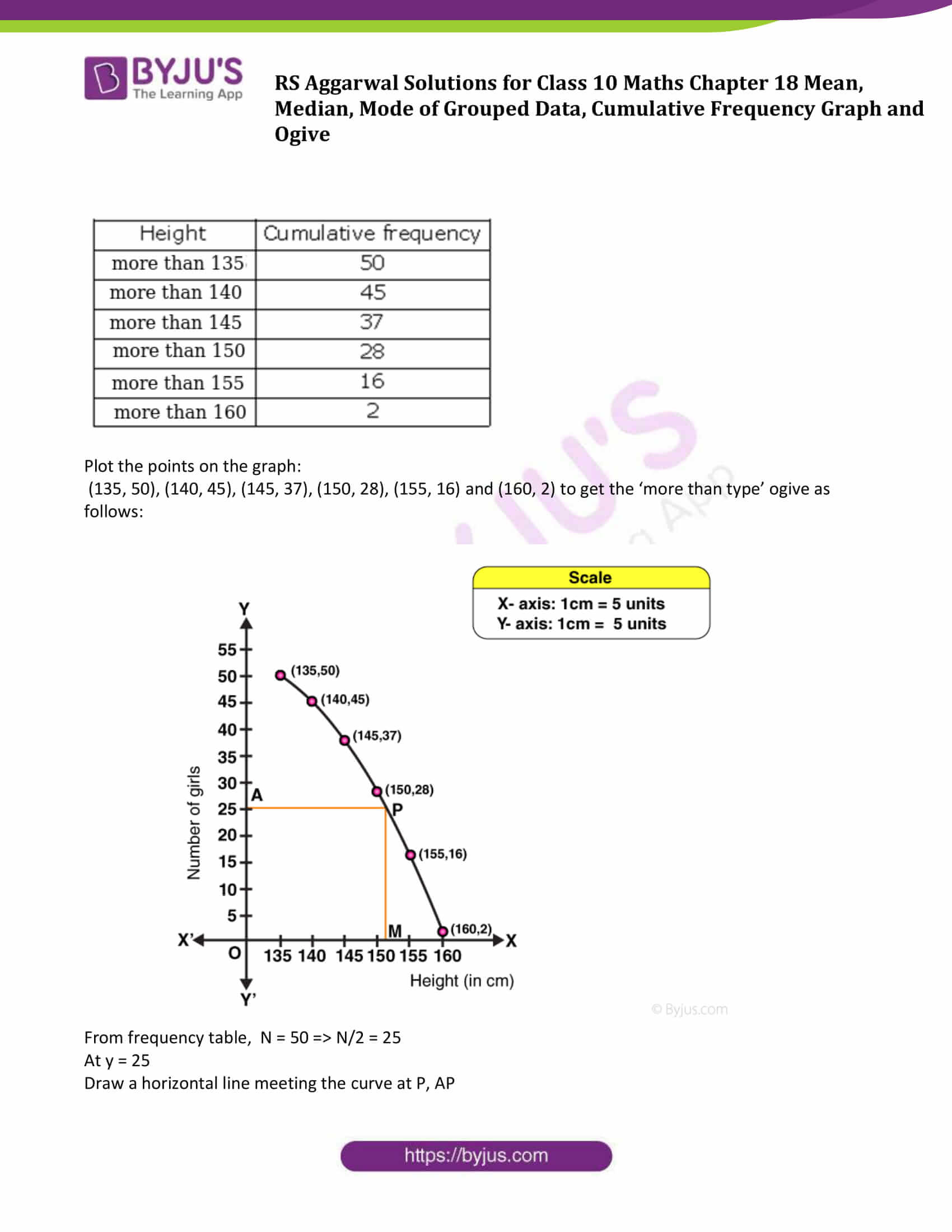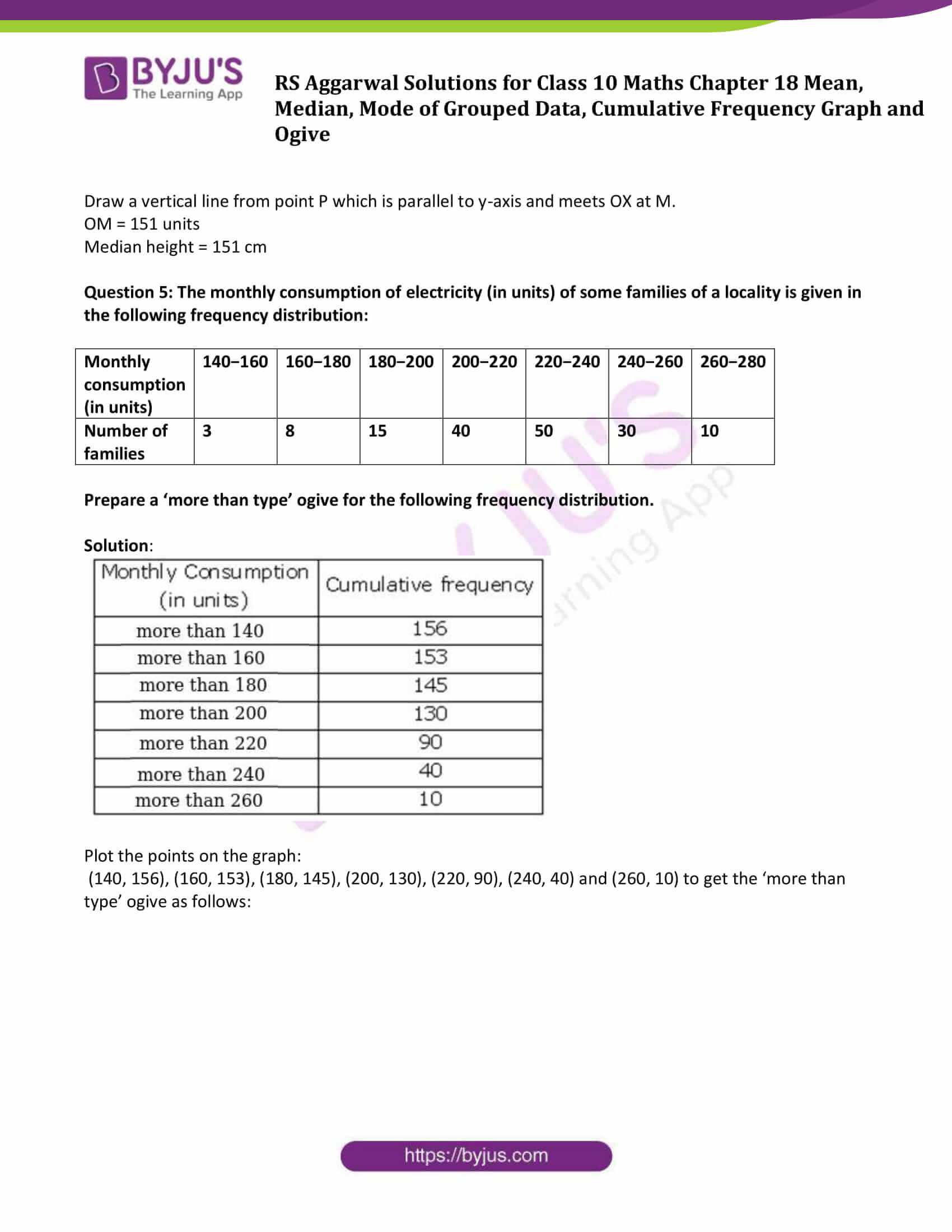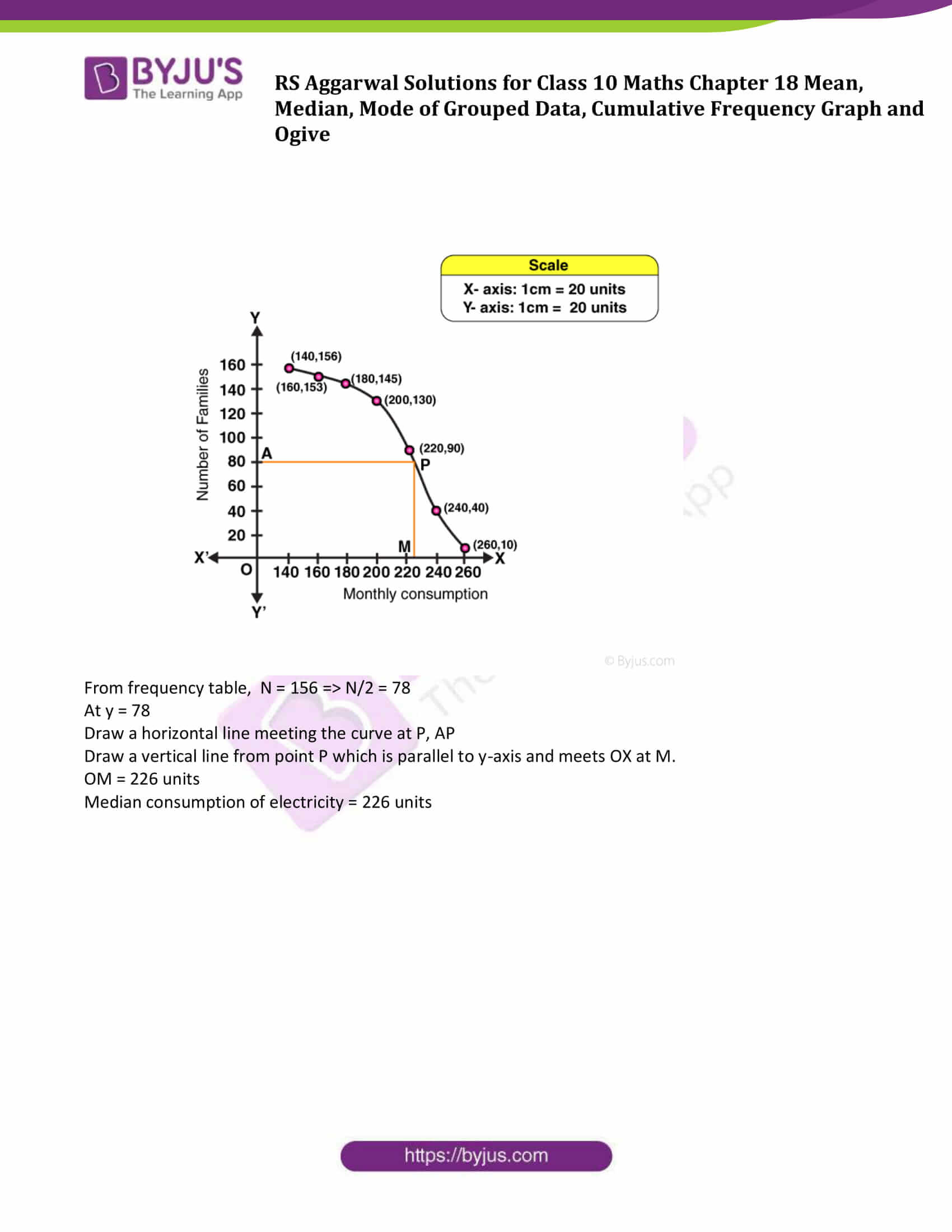## Exercise 18E

Question 1: Find the median of the following data by making a ‘less than ogive’.

 Marks 0−10 10−20 20−30 30−40 40−50 50−60 60−70 70−80 80−90 90−100 Number of students 5 3 4 3 3 4 7 9 7 8

Solution: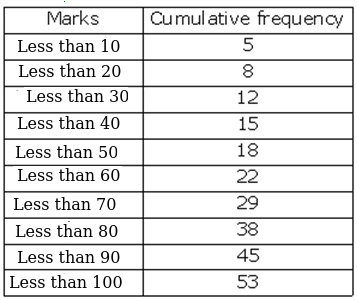Plot points: (10, 5), (20, 8), (30, 12), (40, 15), (50, 18), (60, 22), (70, 29), (80, 38), (90, 45) and (100, 53) to get the ‘less than type’ ogive as follows: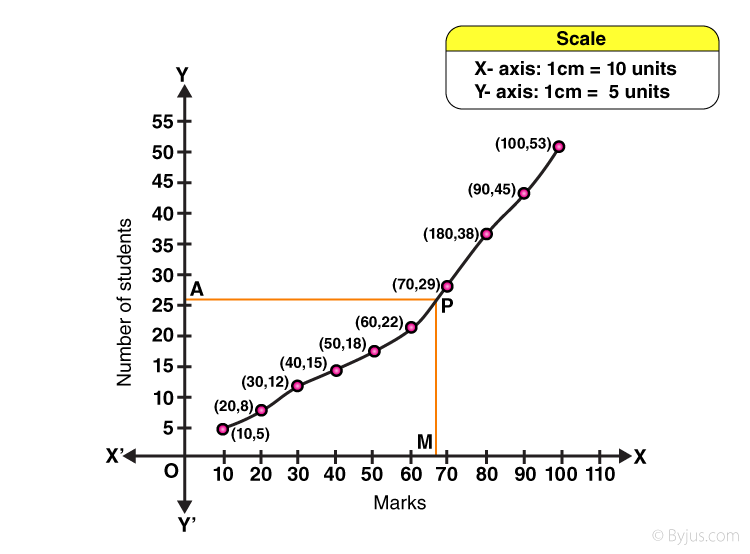From frequency table, N = 53

N/2 = 26.5

Mark y = 26.5 on y-axis

Through A, draw a horizontal line meeting the curve at P.

Through P, a vertical line is drawn which meets OX at M.

OM = 68 units

Hence, median = 68

Question 2: The given distribution shows the number of wickets taken by the bowlers in one-day international cricket matches:

 Number of wickets Less than 15 Less than 30 Less than 45 Less than 60 Less than 75 Less than 90 Less than 105 Less than 120 Number of bowlers 2 5 9 17 39 54 70 80

Draw a ‘less than type’ ogive from the above data. Find the median.

Solution:

Plot points on graph:

(15, 2), (30, 5), (45, 9), (60, 17), (75, 39), (90, 54), (105, 70) and (120, 80) to get the ‘less than type’ ogive as follows: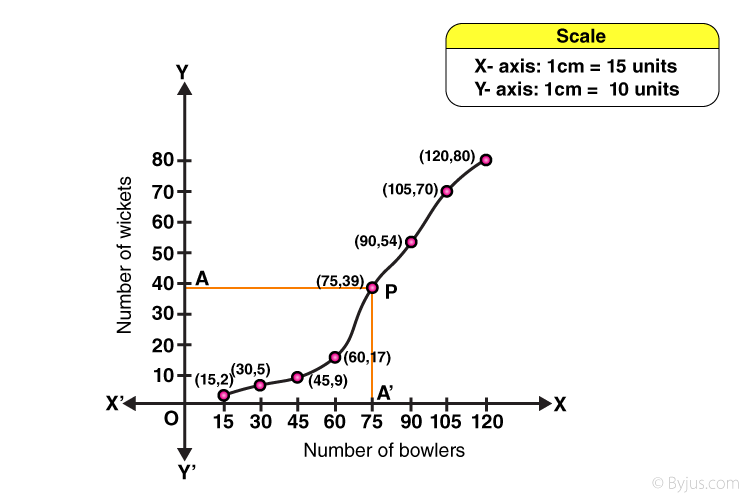From frequency table, N = 80, ⇨ N/2 = 40

At y = 40,

Draw a horizontal line meeting the curve at P, AP

Draw a vertical line from point P which is parallel to y-axis and meets OX at A’.

OM = 77 units

Median number of wickets = 77

Question 3: Draw a ‘more than’ ogive for the data given below which gives the marks of 100 students.

 Marks 0−10 10−20 20−30 30−40 40−50 50−60 60−70 70−80 Number of students 4 6 10 10 25 22 18 5

Solution: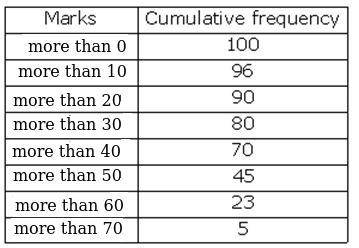Plot points on the graph:

(0, 100), (10, 96), (20, 90), (30, 80), (40, 70), (50, 45), (60, 23) and (70, 5) to get the ‘more than type’ ogive as follows: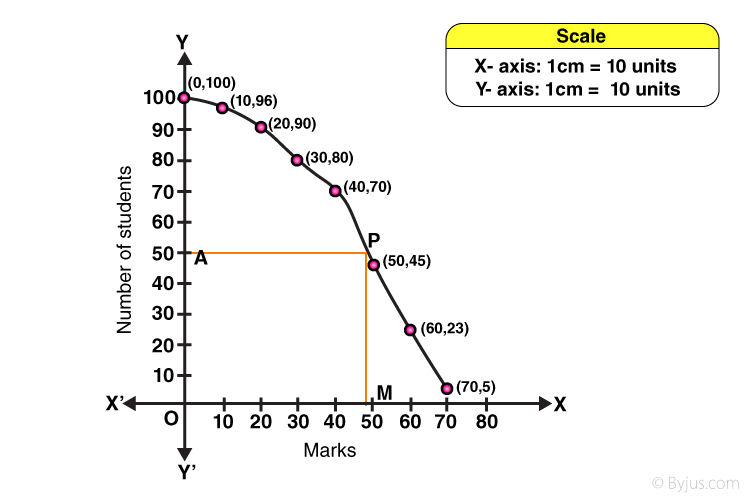From frequency table, N = 100 ⇨ N/2 = 50.

At y = 50

Draw a horizontal line meeting the curve at P, AP

Draw a vertical line from point P which is parallel to y-axis and meets OX at M.

OM = 47 units

Median marks = 47

Question 4:The height of 50 girls of class X of a school are recorded as follows:

 Height (in cm) 135−140 140−145 145−150 150−155 155−160 160−165 Number of girls 5 8 19 12 14 2

Draw a ‘more than type’ ogive for the above data.

Solution: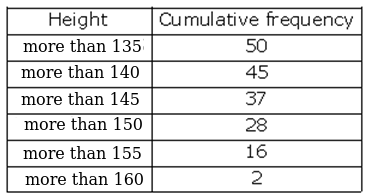Plot the points on the graph:

(135, 50), (140, 45), (145, 37), (150, 28), (155, 16) and (160, 2) to get the ‘more than type’ ogive as follows: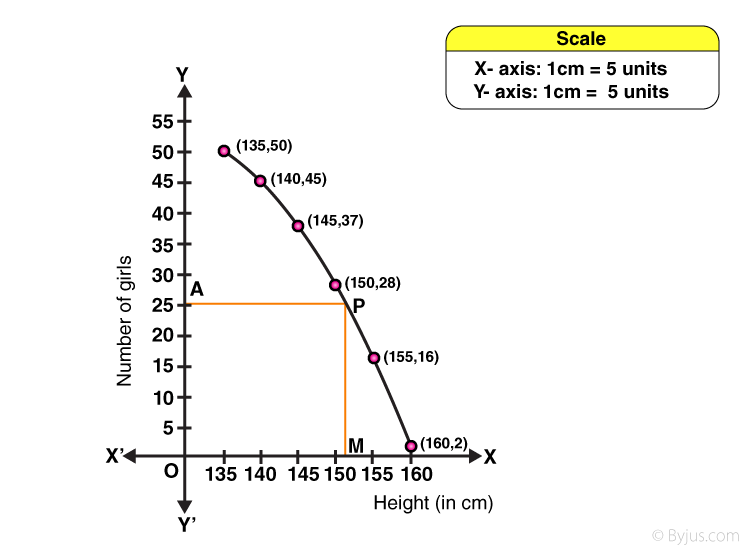From frequency table, N = 50 ⇨ N/2 = 25

At y = 25

Draw a horizontal line meeting the curve at P, AP

Draw a vertical line from point P which is parallel to y-axis and meets OX at M.

OM = 151 units

Median height = 151 cm

Question 5: The monthly consumption of electricity (in units) of some families of a locality is given in the following frequency distribution:

 Monthly consumption (in units) 140−160 160−180 180−200 200−220 220−240 240−260 260−280 Number of families 3 8 15 40 50 30 10

Prepare a ‘more than type’ ogive for the following frequency distribution.

Solution: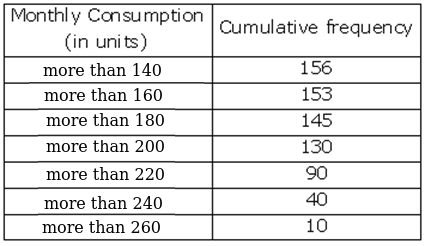Plot the points on the graph:

(140, 156), (160, 153), (180, 145), (200, 130), (220, 90), (240, 40) and (260, 10) to get the ‘more than type’ ogive as follows: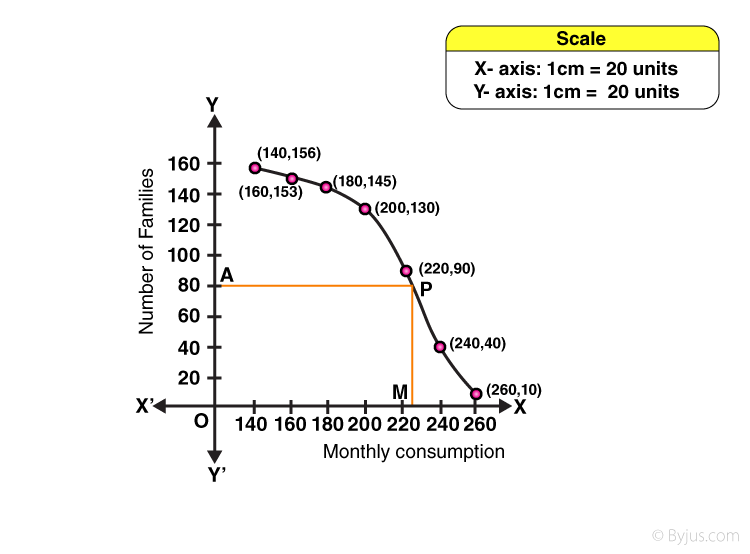From frequency table, N = 156 ⇨ N/2 = 78

At y = 78

Draw a horizontal line meeting the curve at P, AP

Draw a vertical line from point P which is parallel to y-axis and meets OX at M.

OM = 226 units

Median consumption of electricity = 226 units

## Access other exercise solutions of Class 10 Maths Chapter 18 Mean, Median, Mode of Grouped Data, Cumulative Frequency Graph and Ogive

Exercise 18 A Solutions

Exercise 18 B Solutions

Exercise 18 C Solutions

Exercise 18 D Solutions

## R S Aggarwal Solutions for Class 10 Maths Chapter 18 Mean, Median, Mode Exercise 18E Topics:

Class 10 Maths Chapter 18 Mean, Median, Mode of Grouped Data, Cumulative Frequency Graph and Ogive Exercise 18E is based on following topics and subtopics:

• Cumulative Frequency Curve (Ogive)
• Ogive for a ‘less than’ series
• Ogive for a ‘greater than’ series